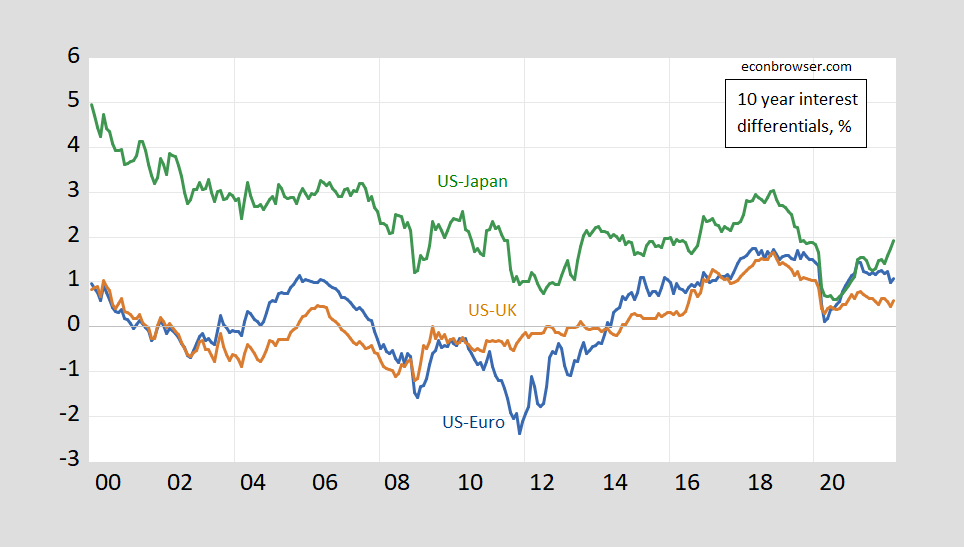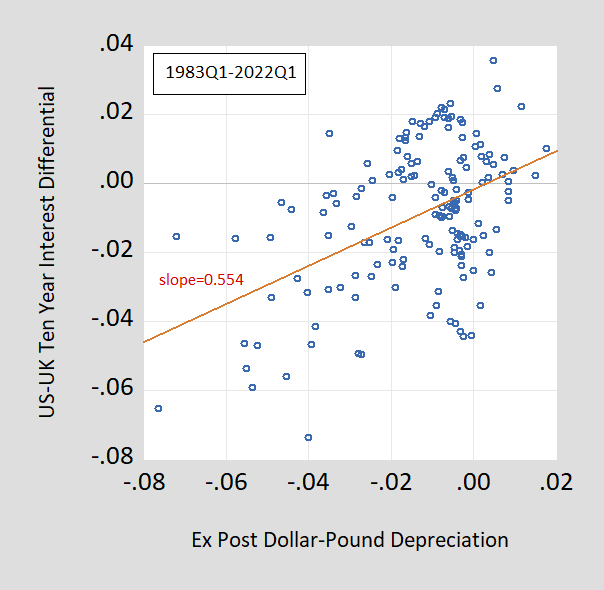### Long Horizon Unbiasedness, UpdatedSome key interest differentials, through March:Figure 1: Ten year government bond yield differential US-euro area (blue), US-UK (brown), US-Japan (green), in %. Source: OECD MEI via FRED, Treasury (for US), and author’s calculations.

As we move away from the zero lower bound (again) and we see more rapid inflation, I thought it interesting to see what linkages might exist between long term interest rates. In particular, will long term rates move in line with expected depreciation rates?

The answer to this question is not really knowable, since we don’t have direct observation on market expectations; and at the 10 year horizon, we don’t really have much survey based expectations data (which contrasts with short horizons like up to a year, as used in Bussiere et al. (2022)). However, we can observe  how subsequent ex post exchange rates moved with interest differentials — up to 2012Q1. What does the past tell us about what to expect.

In Chinn and Meredith (1998), we documented that at longer horizons, interest differentials were positively associated with ex post depreciation. Panel regression coefficients had the following pattern:Figure 2: Panel beta coefficients at different horizons. Notes: up to 12 months, panel estimates for 6 currencies against US\$, euro deposit rates, 1980Q1-2000Q4; 3-year results are zero-coupon yields, 1976Q1-1999Q2; 5 and 10 years, constant yields to maturity, 1980Q1-2000Q4 and 1983Q1-2000Q4 (last observation corresponds to exchange rate data). Source: Chinn (2006).

I updated these results four years ago in this post (and yet earlier, in Chinn and Quayyum (2013). We can evaluate this relationship by using the Fama regression, viz. :

(1)     st+k – st = α + β(itk-itk*) + εt+k

Where s is the log exchange rate defined as home currency units per foreign currency unit (e.g., USD per pound for Americans); itk is the interest rate of maturity remaining k, and * denotes foreign (e.g., UK); and ε is an error term.

The test that the coefficient β is not different from unity is a test for “unbiasedness” — the joint null hypothesis that uncovered interest parity holds and expectations are on average correct (or full information rational expectations hold).

Running the regressions on quarterly data (end-of-period) for the US-UK, one gets:

st+k – st = -0.002 + 0.554(itk-itk*) + εt+k

Adj-R2=0.21, SER= 0.018, DW = 0.018, Nobs = 157, sample 1983Q1-2022Q1. Bold denotes significantly different from value of unity using HAC robust standard errors at the 5% msl.

In this case, with quarterly data, k=40. This regression result summarizes the relationship in this scatterplot:Figure 3: Ten year dollar-pound ex post depreciation annualized against US-UK ten year interest differential lagged 10 years.

For the US-Euro Area:

st+k – st = 0.003 + 0.592(itk-itk*) + εt+k

Adj-R2=0.05, SER= 0.024, DW = 0.011, Nobs = 53, sample 2009Q1-2022Q1. Bold denotes significantly different from value of unity using HAC robust standard errors at the 5% msl.

For US-Japan:

st+k – st = 0.015 + 0.323(itk-itk*) + εt+k

Adj-R2=0.02, SER= 0.032, DW = 0.058, Nobs = 157, sample 1983Q1-2022Q1. Bold denotes significantly different from value of unity using HAC robust standard errors.

In previous studies, Japan has stood out as having a particularly low coefficient. One explanation would be the lack of capital account liberalization in the earlier part of the period. Starting to examine the interest rate data in 1982Q1 (and hence looking at 10 year exchange rate changes beginning in 1992Q1), one obtains:

st+k – st = -0.033 + 1.699(itk-itk*) + εt+k

Adj-R2=0.28, SER= 0.028, DW = 0.161, Nobs = 121, sample 1992Q1-2022Q1. Bold denotes significantly different from value of unity (for slope) or from zero (for constant) using HAC robust standard errors at the 5% msl.

So, the coefficients from a long horizon Fama regression are typically positive (still), and in several cases don’t reject the null of a unit slope coefficient.  That being said, it’s not clear that this correlation is much of a guide to the strength of linkages given the adjusted R2‘s are so low.